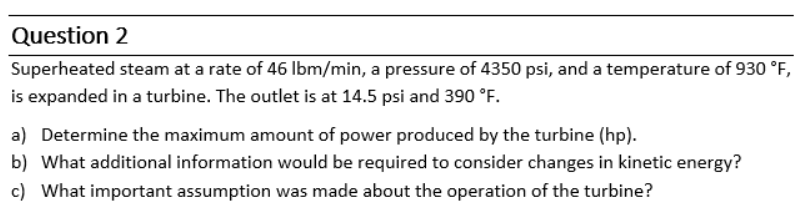# Question Superheated steam at a rate of $$46 \mathrm{lbm} / \mathrm{min}$$, a pressure of $$4350 \mathrm{psi}$$, and a temperature of $$930^{\circ} \mathrm{F}$$, is expanded in a turbine. The outlet is at $$14.5 \mathrm{psi}$$ and $$390^{\circ} \mathrm{F}$$. a) Determine the maximum amount of power produced by the turbine (hp). b) What additional information would be required to consider changes in kinetic energy? c) What important assumption was made about the operation of the turbine?LL8JS6 The Asker · Chemical EngineeringTranscribed Image Text: Superheated steam at a rate of $$46 \mathrm{lbm} / \mathrm{min}$$, a pressure of $$4350 \mathrm{psi}$$, and a temperature of $$930^{\circ} \mathrm{F}$$, is expanded in a turbine. The outlet is at $$14.5 \mathrm{psi}$$ and $$390^{\circ} \mathrm{F}$$. a) Determine the maximum amount of power produced by the turbine (hp). b) What additional information would be required to consider changes in kinetic energy? c) What important assumption was made about the operation of the turbine?
More
Transcribed Image Text: Superheated steam at a rate of $$46 \mathrm{lbm} / \mathrm{min}$$, a pressure of $$4350 \mathrm{psi}$$, and a temperature of $$930^{\circ} \mathrm{F}$$, is expanded in a turbine. The outlet is at $$14.5 \mathrm{psi}$$ and $$390^{\circ} \mathrm{F}$$. a) Determine the maximum amount of power produced by the turbine (hp). b) What additional information would be required to consider changes in kinetic energy? c) What important assumption was made about the operation of the turbine?&#12304;General guidance&#12305;The answer provided below has been developed in a clear step by step manner.Step1/3a) To determine the maximum amount of power produced by the turbine, we need to first calculate the enthalpy of the steam at the inlet and outlet conditions. Then, we can use the mass flow rate and the change in enthalpy to calculate the power produced by the turbine using the following equation:Power = (mass flow rate) * (change in enthalpy)Enthalpy at inlet conditions:From steam tables at 4350 psi and 930&#176;F, the enthalpy of superheated steam is:$$\mathrm{{h}_{{1}}}$$ = 1603.6 Btu/lbmEnthalpy at outlet conditions:From steam tables at 14.5 psi and 390&#176;F, the enthalpy of saturated steam is:hf2 = 343.3 Btu/lbm (saturated liquid enthalpy at 14.5 psi)hg2 = 1195.7 Btu/lbm (saturated vapor enthalpy at 14.5 psi)The steam is superheated at the inlet, so we need to determine the specific enthalpy at the outlet temperature of 390&#176;F using interpolation:h2 = hf2 + x(hg2 - hf2)= 343.3 + x(1195.7 - 343.3)= 1195.7 Btu/lbmwhere x is the quality of the steam, which we can assume to be 1 (i.e., dry steam) since the outlet pressure is close to atmospheric.Power produced by the turbine:Usi ... See the full answer